# Computing Eigenvalues in Extended Precision

by on October 12, 2011

Eigenvalues and eigenvectors play important role in many real-world applications, including control systems modeling, partial differential equations, data mining and clusterization, chemistry, vibration analysis, to name a few examples.

The main practical difficulty is that eigenproblem might be ill-conditioned and hard to compute even when matrix itself is well-conditioned.

This comes from the fact that computing eigenvalues is equivalent to finding roots of a polynomial, which is proven to be unavoidably ill-conditioned problem (even when roots are well-separated, as shown by J.H. Wilkinson [1-3]) .

More precisely, conditioning of an eigenproblem depends on eigenvalues clustering, magnitude and angles between the eigenvectors. All the properties are inherent to the problem and ill-conditioning, if it is present, cannot be easily alleviated or even detected beforehand. The only case when it is considered stable is computing eigenvalues of a symmetric matrix.

At the moment the only reliable way of dealing with ill-conditioned eigenproblems is to use the extended-precision computations. In fact, there is no other alternative in contrast to solving systems of linear equations where various pre-conditioners can be used to improve the conditioning.

Here we want to show two examples of such problems and how toolbox solves them in comparison to MATLAB.

## Example 1. Grcar Matrix

Let’s consider a classic example of sensitive eigenvalues – the Grcar matrix[4-6]. It is composed purely of -1 or 1 elements and has a special structure:

A = gallery('grcar',5) A =   1 1 1 1 0 -1 1 1 1 1 0 -1 1 1 1 0 0 -1 1 1 0 0 0 -1 1

Theory dictates that the Grcar matrix of any dimension has the same eigenvalues of its transpose matrix.

Let’s validate this property by using the standard floating-point arithmetic in MATLAB, and compare it with Multiprecision Computing Toolbox results:

 % MATLAB floating-point precision A = -gallery('grcar',150); plot(eig(A),'k.'), hold on, axis equal plot(eig(A'),'ro')   % Quadruple precision (34 decimal digits) computation. mp.Digits(34); A = mp(-gallery('grcar',150)); plot(eig(A),'k.'), hold on, axis equal plot(eig(A'),'ro')
Results are shown on the plots below. The eigenvalues of the Grcar matrix are displayed in black, and the eigenvalues of the transposed Grcar matrix – in red. In theory they should coincide:Figure 1. MATLAB is unable to generate correct results using standard floating-point precision.Figure 2. Toolbox computes the eigenvalues accurately using the quadruple precision.

Although condition number of the Grcar matrix is low, cond(A) = cond(A') = 3.61, MATLAB’s double precision routines suffer from accuracy loss.

## Example 2. Noschese-Pasquini-Reichel Matrix

Another interesting case is tridiagonal Toeplitz matrices: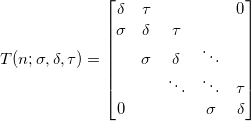Such matrices arise in numerous applications, including the solution of ordinary and partial differential equations, time series analysis, regularization problems, etc. (please check references in  for more details)

Eigenvalues of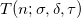lie on a straight line and can be computed explicitly :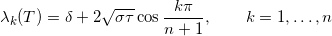As it follows from the expression, transposition doesn’t change the matrix eigenvalues. However, if we apply numerical method to find them and rely on finite precision floating-point arithmetic – accurate solution might be completely lost.

For example, let’s verify the property of eig(T)==eig(T.') for the following matrix :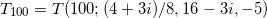% MATLAB standard floating-point precision n = 100; T = toeplitz([16-3i, (4+3i)/8, zeros(1,n-2)], [16-3i, -5, zeros(1,n-2)]); plot(eig(T),'k.'), hold on, axis equal plot(eig(T.'),'ro')   % Multiprecision toolbox (50 decimal digits) computation. mp.Digits(50); n = 100; T = toeplitz([mp('16-3i'),mp('(4+3i)/8'),zeros(1,n-2)], [mp('16-3i'),mp('-5'),zeros(1,n-2)]); plot(eig(T),'k.'), hold on, axis equal plot(eig(T.'),'ro')
As before, the eigenvalues of the initial matrix are displayed in black, and the eigenvalues of the transposed – in red. They should lie on a line segment and coincide with each other:Figure 3. Severe accuracy loss using standard precision in MATLAB.Figure 4. Extended precision computations with 50-digits produce accurate eigenvalues.

The matrix itself is well-conditioned, cond(T) = cond(T.') = 1.81, but double precision results are far off.

The irony here is that to estimate the conditioning of the eigenvalues – we need to compute the eigenvectors , which are even more prone to accuracy loss and require more computational resources:Figure 5. Condition numbers for the eigenvalues of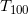, computed with 50-digits of precision by the toolbox (green) and by MATLAB (blue). The difference is almost 30 orders of magnitude!

## Extended-precision routines

Toolbox provides following eigen-functions capable of working with arbitrary precision: eig, condeig, schur, ordeig, ordqz, ordschur, poly, polyeig, qz, hess, balance, cdf2rdf, rsf2csf.

Every function can be used as a drop-in replacement for analogous standard routine, as all support the same options and computation modes. For illustration, all of the following special cases are covered in eig():

Standard eigenproblem: e = eig(A) [V,D] = eig(A) [V,D,W] = eig(A)

Options: [___] = eig(A,balanceOption) [___] = eig(A,B,algorithm) [___] = eig(___,eigvalOption)

Generalized eigenproblem: e = eig(A,B) [V,D] = eig(A,B) [V,D,W] = eig(A,B)

Simple usage example:

 >> mp.Digits(34); % setup working precision   >> A = mp(eye(2)); % convert matrices to extended precision >> B = mp([3 6; 4 8]); % B is singular to make example interesting :)   >> [V,D] = eig(A,B) % solve generalized eigenproblem   V = -0.75 -1 -1 0.5   D = 0.09090909090909090909090909090909092 0 0 Inf   >> A*V(:,1) - D(1,1)*B*V(:,1) % check accuracy of the first eigen-pair   ans = 9.629649721936179265279889712924637e-35 1.925929944387235853055977942584927e-34

Most of the functions are meta-algorithms which analyse the inputs and apply the best matching numerical method for the particular matrix. For example, in case of standard eigenproblem, eig uses multi-shift QR decomposition[10-12] for non-symmetric matrix, MRRR  or divide & conquer algorithm for hermitian, symmetric and tridiagonal symmetric matrices. See this post for more details: Architecture of eigenproblem solver.

## References

1. J. H. Wilkinson (1984). The perfidious polynomial. Studies in Numerical Analysis.
2. J. H. Wilkinson (1959). The evaluation of the zeros of ill-conditioned polynomials. Part I. Numerische Mathematik 1:150-166.
3. J. H. Wilkinson (1963). Rounding Errors in Algebraic Processes. Englewood Cliffs, New Jersey: Prentice Hall.
4. J. F. Grcar. Operator coefficient methods for linear equations. tech. report SAND89-8691, Sandia National Labs, 1989.
5. A. Varga. Solving Control Problems – a Numerical Perspective. IEEE International Symposium on Intelligent Control, 2008.
6. L. N. Trefethen. Psuedospectra of matrices. “Numerical Analysis 1991” (Dundee 1991), Longman Sci. Tech., Harlow, 1992, 234-266.
7. S. Noschese, L. Pasquini, L. Reichel, (2013). Tridiagonal Toeplitz matrices: Properties and novel applications. Numerical Linear Algebra with Applications 20 (2): 302.
8. G. D. Smith, Numerical Solution of Partial Differential Equations, 2nd ed., Clarendon Press, Oxford, 1978.
9. Gene H. Golub and Charles F. Van Loan. Matrix Computations. Johns Hopkins University Press; fourth edition edition (December 27, 2012).
10. Braman K., Byers R., Mathias R., The multi-shift QR algorithm Part I: Maintaining well focused shifts, and level 3 performance, SIAM Journal on Matrix Analysis and Applications, 23:929–947, 2002.
11. Braman K., Byers R., Mathias R., The multi-shift QR algorithm Part II: Aggressive early deflation, SIAM Journal on Matrix Analysis and Applications, 23:948–973, 2002.
12. Kreßner D., Numerical methods and software for general and structured eigenvalue problems, PhD thesis, TU Berlin, Institut für Mathematik, 2004.
13. Dhillon I.S., Parlett B.N., Vömel C., The design and implementation of the MRRR algorithm, ACM Transactions on Mathematical Software 32:533-560, 2006.

Martin March 30, 2012 at 3:28 pm

I think your toolbox sounds very interesting. I have been using the (free) one by Ben Barrowes until now and I adapted it to Windows so I could use it at work, BUT, I am not really happy about the SVD function–which relates to eigenvalues and that is why I posted here. How is “your” SVD implemented for multiple precision. Is it like the MATLAB function “svd.m” with all its special cases included, or is is more like the one in Octave?

Pavel Holoborodko March 30, 2012 at 10:41 pm

Thank you for your comment. We do support all special cases of svd, such as:
 s = svd(X) [U,S,V] = svd(X) [U,S,V] = svd(X,0) [U,S,V] = svd(X,'econ')

Our main goal is to make multiple precision functions to be one-to-one equivalent for built-in routines in Matlab, so that all special cases and features are also available in multiple precision.

Internally we use current state-of-the-art divide & conquer algorithm for computing SVD. It is implemented in C++ with optimizations for multi-core architectures. Please check this post for more references on algorithm: http://www.advanpix.com/2015/02/12/version-3-8-0-release-notes/

Rui September 24, 2013 at 1:01 pm

Is there a linux version that I can install? Thanks!

Pavel Holoborodko September 24, 2013 at 1:16 pm

Antonio April 1, 2014 at 2:13 am

I’m looking for a Mac OS X version. Do you have one available? Thanks!

Pavel Holoborodko April 1, 2014 at 2:38 am

Dr. Wajdi Gaddah January 26, 2014 at 5:51 pm

I need to know how the Multiprecision Computing Toolbox can be applied to eigs() function

Pavel Holoborodko January 27, 2014 at 7:49 am

Dr. Wajdi,

Thank you for your question. At the moment “eigs” is not implemented in toolbox.
We will add it in future versions if we get enough requests for it.

Dr. Wajdi Gaddah January 27, 2014 at 9:04 pm

Dear Pavel

Thank you for your reply. I would like to let you know that The MATLAB function eigs is very important in computational physics and engineering, because it is the only command in MATLAB that is capable of solving large scale Hermitian, non-Hermitian, symmetric or nonsymmetric, standard or generalized sparse matrix eigenvalue problems from significant application areas. I hope you add it in the Multiprecision Computing Toolbox in the near future. I will definitely buy the software if the eigs function is included in the future version.

Enrico Onofri December 16, 2016 at 6:00 pm

I agree that eigs is a fundamental tool for technical as well as in pure research. I use it in computing
energy spectra for quantum Hamiltonians up to three dimensions. Its ability to accept a routine which defines the action of the operator instead of providing its matrix representation is very effective in implementing a spectral representation of the Laplacian through fft with remarkable accuracy as compared to finite differences. I hope eigs will be implemented in the near future in advanpix.

Pavel Holoborodko December 17, 2016 at 4:57 am

Dear Enrico,

Thank you very much for detailed request. I have been receiving requests for EIGS from many other researchers. And, in fact, we are working on it right now – plan to include it in next version.

EIGS has many special cases – please let me know what mode you are interested in (‘LM’, ‘SM’, standard or generalized form, shift if any, etc.). I will send you the pre-release version of EIGS if it covers your case.

We use Krylov-Schur with (aggressive) deflation. There are many things to improve (speed of re-orthogonalization, etc.) but it already shows good performance compared to Arnoldi with implicit restarts (ARPACK) – the algorithm behind MATLAB’s built-in EIGS.

Also I would appreciate if you would share (brief) example code, so that we can test your case and improve the algorithm if required. Thank you.

Robert Jones August 2, 2018 at 9:09 pm

Interesting I only came across this now. I also found that multi-precision is the “trick” that one uses to deal with ill-conditioning. I first saw it in 1993, but as a student of Physics, didn’t know “ill-conditioning” by name, but I didn’t let that bother me. There are other methods to get around ill-conditioning (to solve the problem), but simply working in higher precision seems far easier.

FuCheng October 26, 2021 at 3:42 pm

I want to know if the eigs() function is added in the current version, especially the “largestreal” mode.